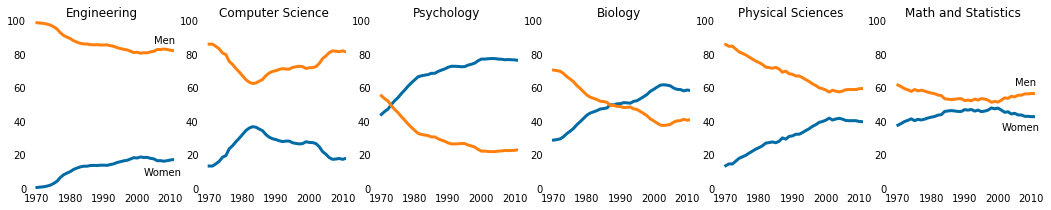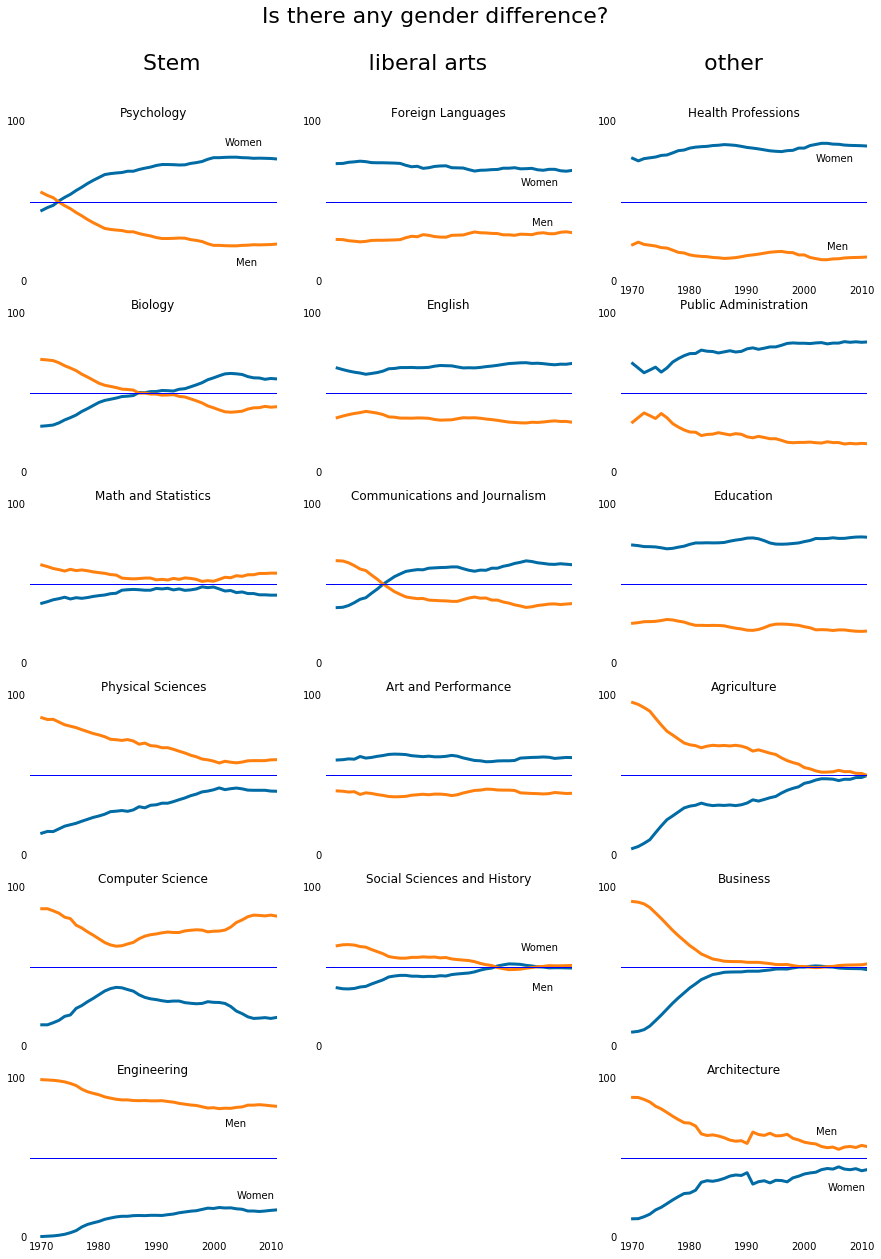In :
%matplotlib inline
import pandas as pd
import matplotlib.pyplot as plt

cb_dark_blue = (0/255,107/255,164/255)
cb_orange = (255/255, 128/255, 14/255)
stem_cats = ['Engineering', 'Computer Science', 'Psychology', 'Biology', 'Physical Sciences', 'Math and Statistics']

fig = plt.figure(figsize=(18, 3))

for sp in range(0,6):
ax.plot(women_degrees['Year'], women_degrees[stem_cats[sp]], c=cb_dark_blue, label='Women', linewidth=3)
ax.plot(women_degrees['Year'], 100-women_degrees[stem_cats[sp]], c=cb_orange, label='Men', linewidth=3)
for key, spine in ax.spines.items():
spine.set_visible(False)
ax.set_xlim(1968, 2011)
ax.set_ylim(0,100)
ax.set_title(stem_cats[sp])
ax.tick_params(bottom="off", top="off", left="off", right="off")

if sp == 0:
ax.text(2005, 87, 'Men')
ax.text(2002, 8, 'Women')
elif sp == 5:
ax.text(2005, 62, 'Men')
ax.text(2001, 35, 'Women')
plt.show()Because there are seventeen degrees that we need to generate line charts for, we'll use a subplot grid layout of 6 rows by 3 columns. We can then group the degrees into STEM, liberal arts, and other, in the following way:

In [ ]:
stem_cats = ['Psychology', 'Biology', 'Math and Statistics', 'Physical Sciences', 'Computer Science', 'Engineering']
lib_arts_cats = ['Foreign Languages', 'English', 'Communications and Journalism', 'Art and Performance', 'Social Sciences and History']
other_cats = ['Health Professions', 'Public Administration', 'Education', 'Agriculture','Business', 'Architecture']
cats=[stem_cats,lib_arts_cats,other_cats]


Concept is STEM category takes the value of 1, 4, 7, 10, 13, 16 as subplot reference, Liberal_Arts category takes the value 2, 5, 8, 11, 14, 17 and Other Category takes the values 3, 6, 9, 12, 15, 18 Since SP starts at 0 ends at 18(excluded) and is increased by 3, SP+1 starts at 0 or 1 or 2, ends at 19(excluded), and is increased by 3.

In :
#using colorblind friendly colors
cb_light_blue = (162/255, 200/255, 236/255)
cb_light_orange = (255/255, 188/255, 121/255)

#plot

fig = plt.figure(figsize=(15, 20))
for i in range(0,3): # for 3 columns
for j in range(len(cats[i])): # for different counts in cats
ax.plot(women_degrees['Year'], women_degrees[cats[i][j]], c=cb_dark_blue, label='Women', linewidth=3)
ax.plot(women_degrees['Year'], 100-women_degrees[cats[i][j]], c=cb_orange, label='Men', linewidth=3)
for key, spine in ax.spines.items():
spine.set_visible(False)
ax.set_xlim(1968, 2011)
ax.set_ylim(0,100)
ax.set_title(cats[i][j])
ax.tick_params(bottom='off', top='off', left='off', right='off',labelbottom='Off')
ax.set_yticks([0,100]) # set y label to 0 and 100 only

ax.axhline(50) # set horizontal line in chart

#annotate "Women" and "Men"
if (i == 0 and j == 0):
ax.text(2002, 85, 'Women')
ax.text(2004, 10, 'Men')

if (i == 0 and j == 5):
ax.text(2002, 70, 'Men')
ax.text(2004, 25, 'Women')
ax.tick_params(labelbottom='on')

if (i == 1 and j == 0):
ax.text(2002, 60, 'Women')
ax.text(2004, 35, 'Men')

if (i == 1 and j == 4):
ax.text(2002, 60, 'Women')
ax.text(2004, 35, 'Men')

if (i == 2 and j == 0):
ax.text(2002, 75, 'Women')
ax.text(2004, 20, 'Men')
ax.tick_params(labelbottom='on')

if (i == 2 and j == 5):
ax.text(2002, 65, 'Men')
ax.text(2004, 30, 'Women')
ax.tick_params(labelbottom='on')
#set title of figure
fig.suptitle("Is there any gender difference?" + "\n\n"+"     Stem"+ "                        liberal arts"
+ "                               other", fontsize=22)

# export the file, need to be called before showing it
plt.savefig('gender_gap.png')
plt.show()In Stem, gap between Men and Women has reduced but less than Liberal arts and other majors

In [ ]: